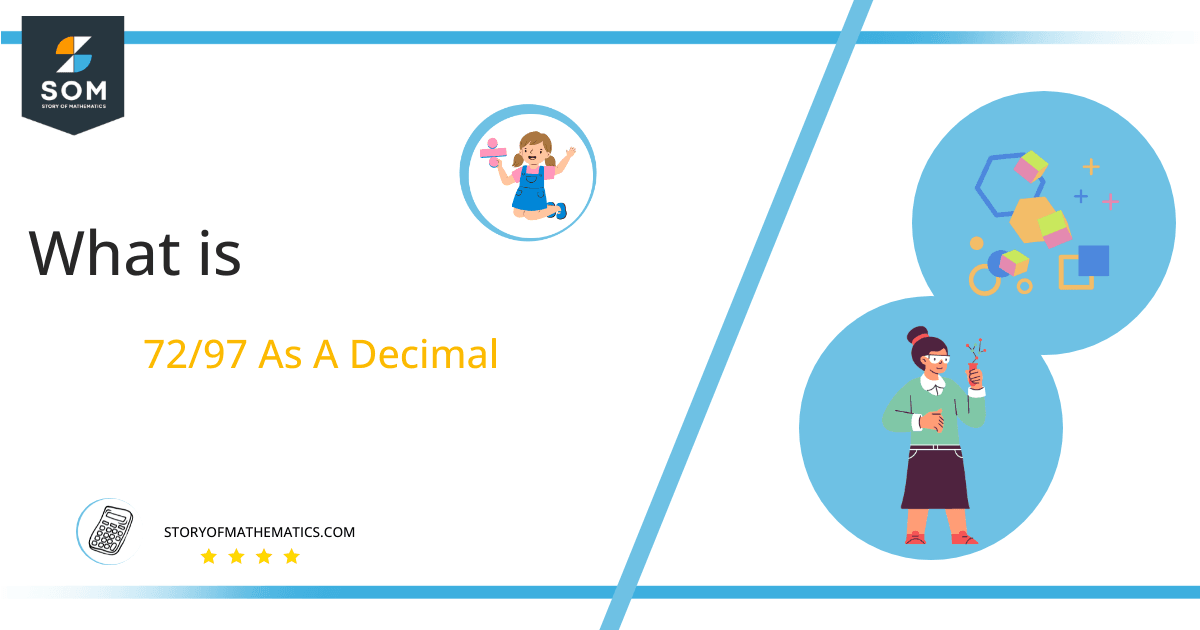# What Is 72/97 as a Decimal + Solution With Free Steps

The fraction 72/97 as a decimal is equal to 0.742.

The division of two numbers p and q is usually notated as $\boldsymbol\div$ q, where p is the dividend and q is the divisor. Sometimes, we show this in the form of a fraction p/q, which is easier and faster to write. Here, p is called the numerator and q is called the denominator.Here, we are more interested in the division types that result in a Decimal value, as this can be expressed as a Fraction. We see fractions as a way of showing two numbers having the operation of Division between them that result in a value that lies between two Integers.

Now, we introduce the method used to solve said fraction to decimal conversion, called Long Division, which we will discuss in detail moving forward. So, let’s go through the Solution of fraction 72/97.

## Solution

First, we convert the fraction components, i.e., the numerator and the denominator, and transform them into the division constituents, i.e., the Dividend and the Divisor, respectively.

This can be done as follows:

Dividend = 72

Divisor = 97

Now, we introduce the most important quantity in our division process: the Quotient. The value represents the Solution to our division and can be expressed as having the following relationship with the Division constituents:

Quotient = Dividend $\div$ Divisor = 72 $\div$ 97

This is when we go through the Long Division solution to our problem.Figure 1

## 72/97 Long Division Method

We start solving a problem using the Long Division Method by first taking apart the division’s components and comparing them. As we have 72 and 97, we can see how 72 is Smaller than 97, and to solve this division, we require that 72 be Bigger than 97.

This is done by multiplying the dividend by 10 and checking whether it is bigger than the divisor or not. If so, we calculate the Multiple of the divisor closest to the dividend and subtract it from the Dividend. This produces the Remainder, which we then use as the dividend later.

Now, we begin solving for our dividend 72, which after getting multiplied by 10 becomes 720.

We take this 720 and divide it by 97; this can be done as follows:

720 $\div$ 97 $\approx$ 7

Where:

97 x 7 = 679

This will lead to the generation of a Remainder equal to 720 – 679 = 41. Now this means we have to repeat the process by Converting the 41 into 410 and solving for that:

410 $\div$ 97 $\approx$ 4

Where:

97 x 4 = 388

This, therefore, produces another Remainder which is equal to 410 – 388 = 22. Now we must solve this problem to Third Decimal Place for accuracy, so we repeat the process with dividend 220.

220 $\div$ 97 $\approx$ 2

Where:

97 x 2 = 194

Finally, we have a Quotient generated after combining the three pieces of it as 0.742, with a Remainder equal to 26.Images/mathematical drawings are created with GeoGebra.